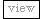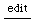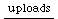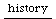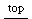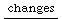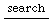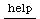Back to Theory

## "Discrete" and "continuous" computation of objects in SC 2

(UGen graph computation and handling in SC2 is similar in SC3)
Audio rate and control rate
a crucial point

related topic: evaluation dynamics of functions | Understanding Assignment

Let us examine how arguments for a sound function (a function that produces a signal and can be used as argument for Synth) are created. We distinguish two kinds of objects which can be arguments of a sound functions:

1. Discrete objects such as numbers, symbols, arrays - including Signal arrays when these are not parts of a input signal.
Examples: ...
2. Continuous objects, i.e. objects which create audio-rate or control-rate signals that are evaluated continuously as inputs within the sound function to which they are passed as arguments. These are basically all UGens or connected groups of UGens forming "UGen graphs". Because such objects create signals, their evaluation is different from the evaluation of a discrete object. While a discrete object is evaluated only once to obtain its value, continuous objects generate a stream of values which can be of indefinite length. Moreover they need to produce this stream of values at a steady rate in order to generate digital audio output. This generation of digital output is done by instances of the class "Synth". These instances work by connecting "continuous" UGen objects with other objects to form UGen graphs which are continuously evaluated as long as the Synth instance they are part of produces a signal. "Continuously" means here that the object is computed repeatedly at a constant interval which is determined by the audio sampling rate and a given block size. At each block-interval the Synth instance computes an array of values equal to the blocks size, which is a stream of digital audio samples (for multichannel audio, an array of such streams is computed).

Audio rate and control rate unit generators are computed differently within the synth: Audio rate unit genrators are computed once for each sample of the stream. Control-rate unit generators are computed only once for each block, and their values are interpolated to audio rate when they are combined with audio-rate unit generators.
Examples:...

As a consequence of the way Synth works, functions that return "continuous objects" only work when they are evaluated within a running Synth instance, because the Synth needs to "hardwire" their components to create a unit generator graph capable of continuous evaluation. In other words, a unit generator or a graph ("combination") of unit generators will only work if it is returned from a function which is evaluated inside a synth instance. For example consider following pairs of correct and wrong code:

#### Example 1.

```Synth.play( { FSinOsc.ar(400, 0.1) } ) // correct: Synth takes a function as argument { }, the
// function returns a unit generator which the Synth installs for continuous evaluation.

Synth.play(FSinOsc.ar(400, 0.1)) // wrong: a unit generator cannot be installed in a synth,
// unless it is returned by a function passed to the synth as argument.
```

#### Example 2.

Both examples below are incorrect: "You must create the FSinOsc within the Synth's function argument. Otherwise it has no parent Synth and never gets run. " (JMcC. 21.2.2001)

```var ugen;
ugen = FSinOsc.ar(400, 0.1);  // store a unit generator in a variable;
Synth.play( { ugen });  //
```

But:

```var ugen;
ugen = FSinOsc.ar(400, 0.1);
Synth.play( ugen ); //
```

#### Example 3.

```var ugenfunc;
ugenfunc = { FSinOsc.ar(400, 0.1) }; // define a UGen making function.
Synth.play(ugenfunc);           // correct: pass the function as argument to Synth.
```

## Using functions which generate UGen graphs as arguments to other sound functions

Next we take example 3 above one step further: We define a function which takes arguments for values of the parameters that govern its sounds behavior.
Consider for example a tone generated by a sine oscillator (SinOsc) as unit generator. The SinOsc unit generator has three parameters: frequency, phase and amplitude. A sound function for generating a tone with SinOsc might thus have three arguments for setting the values of these parameters:

```soundfunc = { arg freq, phase, amp; SinOsc.ar(freq, phase, amp) }
```

To give the parameters freq, phase, and amp specific numerical values we need to evaluate the function soundfunc with numbers as arguments:

```soundfunc.value(400, 0, 0.2)
```

will give freq the value 400, phase the value 0 and amp the value 0.2. Yet even though soundfunc is a function, putting the above statement alone inside a synth to play will result in an error:

```Synth.play( soundfunc.value(400, 0, 0.2) );  // Error ...
```

Why is this? To understand exactly why the example above produces an error we must analyze what happens when the example code is evaluated. The components of the example are as follows:

```Synth :                           A class object (the Synth class).
play:                                   a message to be passed to the Synth class object.
soundfunc.value(400, 0, 0.2):   an argument associated with the message play.
```

The crucial point in this example is that expressions which form parts of arguments passed to a function are evaluated before the function, and the result of their evaluation is then passed as actual value of the argument to the function. Thus, what is passed to Synth.play(...) is not the function soundfunc, but the result of its evaluation. The result of evaluating soundfunc is not a function but a UGen (SinOsc), as can be seen by evaluating the expression on its own with Command-P or by appending the message .postln to print the result:

```soundfunc.value(400, 0, 0.2).postln;  // posts ....
```

Since for the reason already explained, Synth.play(....) can only take a function as first argument, giving it a UGen instead of a function will produce an error.

The correct

```Synth.play( {soundfunc.value(400, 0, 0.2) } );
```

Note that we have to enclose the evaluation within another function, because the Synth needs a function from which it internally creates a signal-generating graph to play. Thus, evaluating the soundfunc by itself in the Synth - without an enclosing function - will generate an error:

```Synth.play( soundfunc.value(400, 0, 0.2) );
```

The above example functions well when the parameters passed to soundfunc are discrete objects such as numbers. If however, ...